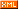# 不再为生存而代码

 博客园 :: 首页 :: 博问 :: 闪存 :: 新随笔 :: 联系 :: 订阅:: 管理 ::Javascript中Math对像的一些复杂算术运算方法:

Math.pow(2,53)  //2的53次幂 结果：9007199254740992

Math.round(0.6)  //0.6四舍五入 结果：1.0

Math.ceil(0.6)　　//0.6向上求整 结果：1.0

Math.floor(0.6) 　　//0.6向下求整 结果：0

Math.abs(-5)　　　　//-5求绝对值 结果：5

Math.max(x,y,z)　　//返回 x,y,z中最大的一个

Math.min(x,y,z)　　//返回 x,y,z中最小的一个

Math.random()　　//生成一个大于等于0小于1.0的伪随机数

Math.PI　　　　　　//常数圆周率

Math.E　　　　　　//e:自然对数的底数

Math.sqrt(3)　　　　//3的平方根

Math.pow(3,1/3)　　//3的立方根

Math.sin(0)　　　　//三角函数 正弦函数

Math.cos(0)　　　　//三角函数  余弦函数

Math.atan(0)　　　　//三角函数

Math.log(10)　　　　//10的自然对数

Math.log(100)/Math.LN10　　//以10为底100的对数

Math.log(512)/Math.LN2　　　//以2为底512的对数

Math.exp(3)　　　　//e三次幂

posted on 2016-05-18 09:14  贤勇  阅读(455)  评论(0编辑  收藏  举报